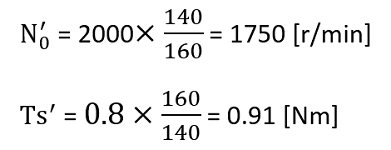1. TOP
2. 五十嵐電機製作所コラム
3. en_Article en motor info

## What is “Motor Performance Chart”?

### This performance chart is written in the torque reference system.

Based on torque T [Nm],
you can read the following parameters;

Rotation speed  : N [r / min]

Current   : I [A]

Output   : P [W]

Efficiency   : η [%]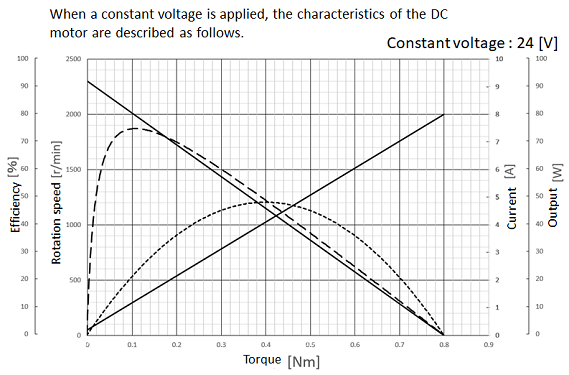Torque is the moment of force around the axis of rotation and represents the rotational force of the motor.

The unit is [Nm].

## How to read “Motor Performance Chart”

### Torque - Rotation

Horizontal axis  : Torque [Nm]

Vertical axis  : Rotation speed [r / min]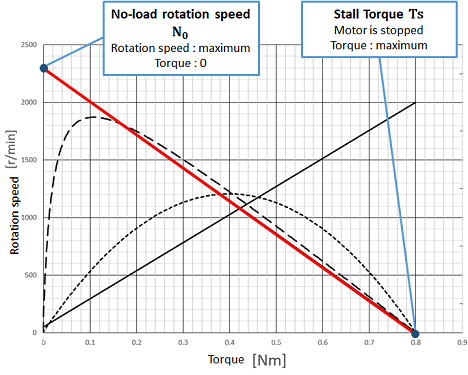Rotation speed when the motor is not loaded

Stall torque Ts [Nm]

Torque when the load of the motor is increased and the rotation of the motor is stopped

Examples from the right figure

N0＝2300 [r/min]

Ts ＝   0.8 [Nm]

### Torque - Current

Horizontal axis  : Torque [Nm]

Vertical axis  : Current [A]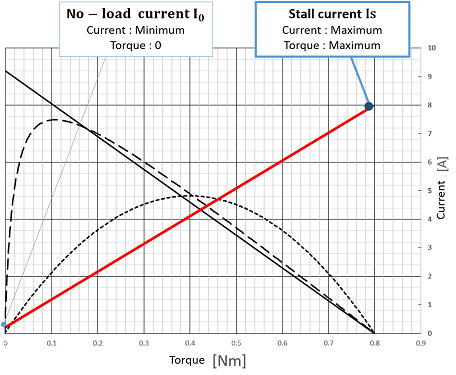Current that flows when a voltage is applied when the motor is not loaded

Stall current Is [A]

Current when the load of the motor is increased and the rotation of the motor is stopped

Examples from the right figure

I0 ＝ 0.2 [A]

Is ＝ 8.0 [A]

### Torque – Output

Horizontal axis  : Torque [Nm]

Vertical axis  : Output [W]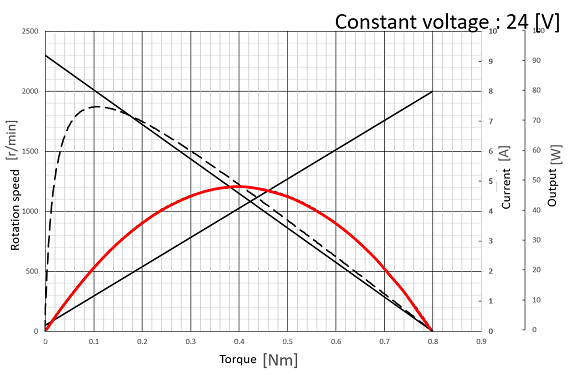Output

P [W] = N [rad/sec] × T [Nm]

= N [r/min] × 2π/60  × T [Nm]

The output line can be drawn from the rotation line.

Example:  Output at 0.1 [Nm]

2000[r/min] × 2π/60 × 0.1[Nm] = 21[W]

### The efficiency line

Horizontal axis  : Torque [Nm]

Vertical axis  : Efficiency [%]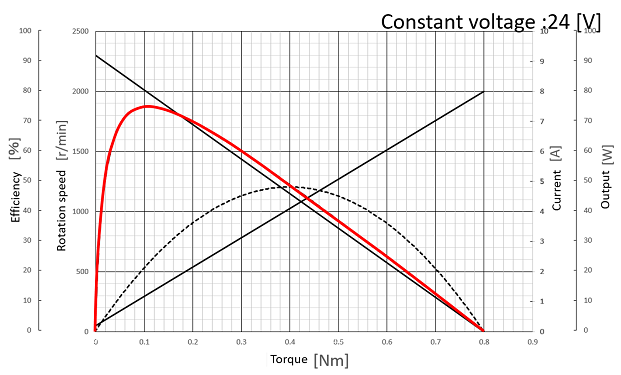Efficiency

η [％] = (P [W])/((V × I)  [W])  × 100

The efficiency line can be drawn from the current line and applied voltage.

Example :  Efficiency  at 0.1 [Nm]

21/(24×1.2) ×100 = 73[％]

## Changes in each parameter

### Changing the following four parameters will affect the performance chart.

Voltage of power-supply

Change in voltage applied to the motor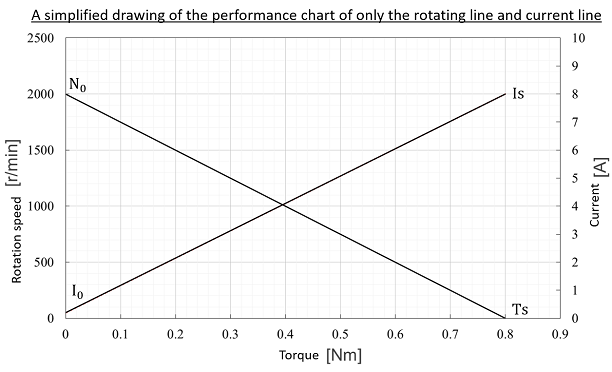Number of turns

Change in the number of turns of the winding wound around the core

Diameter of winding wire

Change in wire diameter of the winding wound around the core

Type of magnet

Change in magnetic force

#### Voltage of power-supply

If only the voltage of power-supply changes, the performance is proportional to the change of the voltage of power-supply.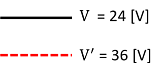V ：Original voltage

V′：Voltage after changed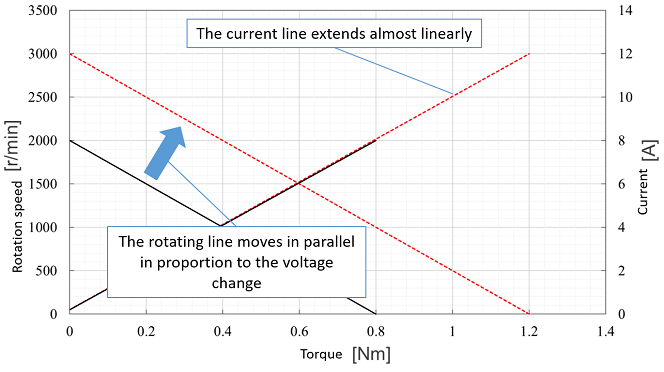N0′ = N0×V′/V
Is′ = Is×V′/V
Ts′ = Ts×V′/V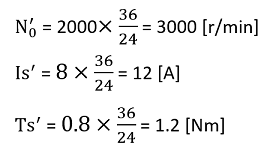#### Number of turns

When the number of turns is reduced, the no-load rotation speed and stall current increase proportionally.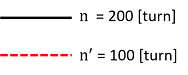n ：original number of turns

n′：number of turns after changedN0′ = N0×n/n′
I0′= I0 × n/n′
Is′ = Is×n/n′
Ts′ = Ts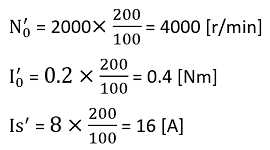#### Diameter of winding wire

When the diameter of winding wire is increased, the stall torque and stall current change in proportion to the square of the diameter of winding wire.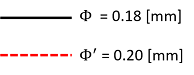Φ：original diameter of winding wire

Φ′： diameter of winding wire after changed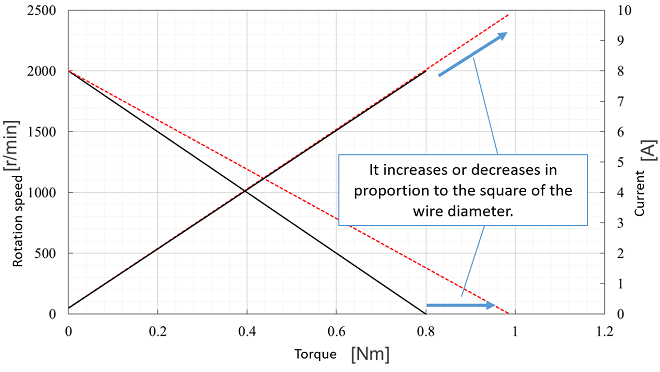N0′ = N0
Is′ = Is×(  Φ′/Φ  )2
Ts′ = Ts ×(  Φ′/Φ  )2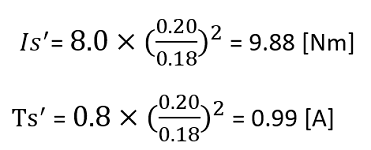#### Type of magnet

Performance changes as the strength of the magnetic force changes.

The dry type is weaker and the wet type is stronger.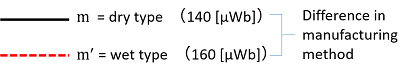N0′ = N0 × m/m′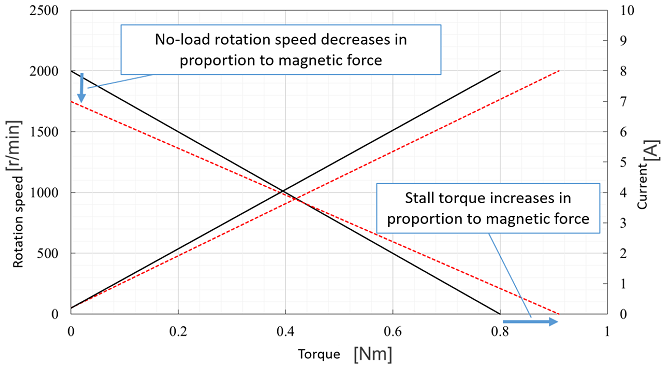Is′ = Is
Ts′ = Ts ×  m′/m